# Calculating Skewness and Kurtosis in PythonSkewness is a statistical measure of asymmetric distribution of data while kurtosis helps determine if the distribution is heavy-tailed compared to a normal distribution.

The most common type of data and probability distribution is a normal distribution. It is defined by a symmetric bell-shaped curve.

Normal distribution can become distorted under significant causes. It is calculated using skewness and kurtosis, which this article will explore in detail with respect to Python.

## Normal distribution

A continuous distribution of random values is called a normal distribution. A random value is one that depends on the outcome of a random event. For example, you either get heads or tails when you flip a coin. But you cannot determine with certainty what you will get.

When you’re plotting against something that has only a probable chance of happening, you will get a probability distribution. The probability of random values that can take on a value is known as a continuous probability distribution.

The number of values that the probability has are infinite and will form a continuous curve. So, instead of writing the probability variables, you can define the range in which they lie.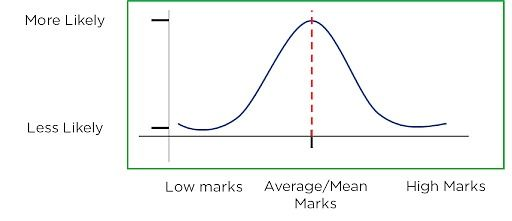Image source

When the continuous probability distribution curve is bell-shaped like a hill with a well-defined peak, it is a normal distribution. The peak should be at the mean and the data must be symmetrically distributed on both sides. The median, mode, and mean are equal and lie closer together.

## Skewness

Skewness is a way of estimating and measuring the shape of a distribution. It is a vital statistical method for estimating asymmetrical behavior rather than computing the frequency distribution. Its value can be either positive or negative.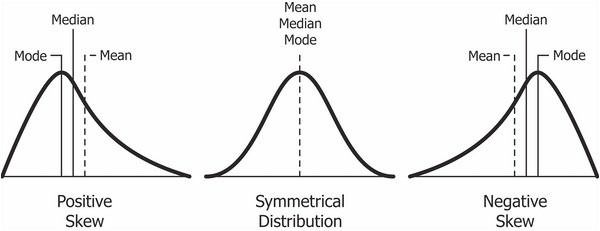Image source

A positive skew will indicate that the tail is on the right side. It will extend toward the most positive values.
On the other hand, a negative skew will indicate a tail on the left side and will extend to the more negative side.
A zero value will indicate that there is no skewness in the distribution, which means that the distribution is perfectly symmetrical.

The distribution of skewness values is as below:

• Skewness = 0 when the distribution is normal.
• Skewness > 0 or positive when more weight is on the left side of the distribution.
• Skewness < 0 or negative when more weight is on the right side of the distribution.

### Calculating skewness

Skewness is mostly calculated using the Fisher-Pearson Coefficient of Skewness. However, there are many more ways to calculate it such as Kelly’s Measure, Bowley, and Momental.

Skewness looks at the measure of skewness as the third standard moment of distribution. It might seem daunting to understand at first, but it will become easier when you learn the steps below.

The Kth moment of a distribution is calculated as: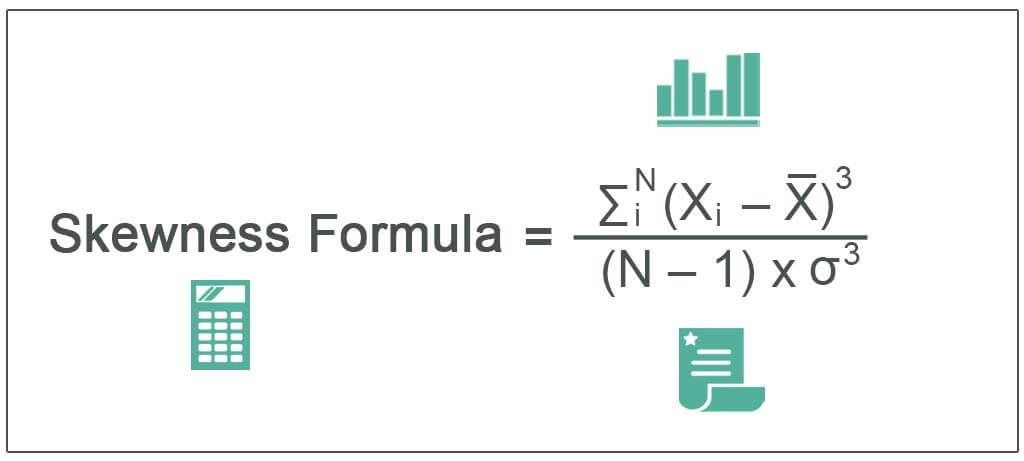Image source

To correct for statistical bias, you need to solve the adjusted FP standardized moment coefficient as: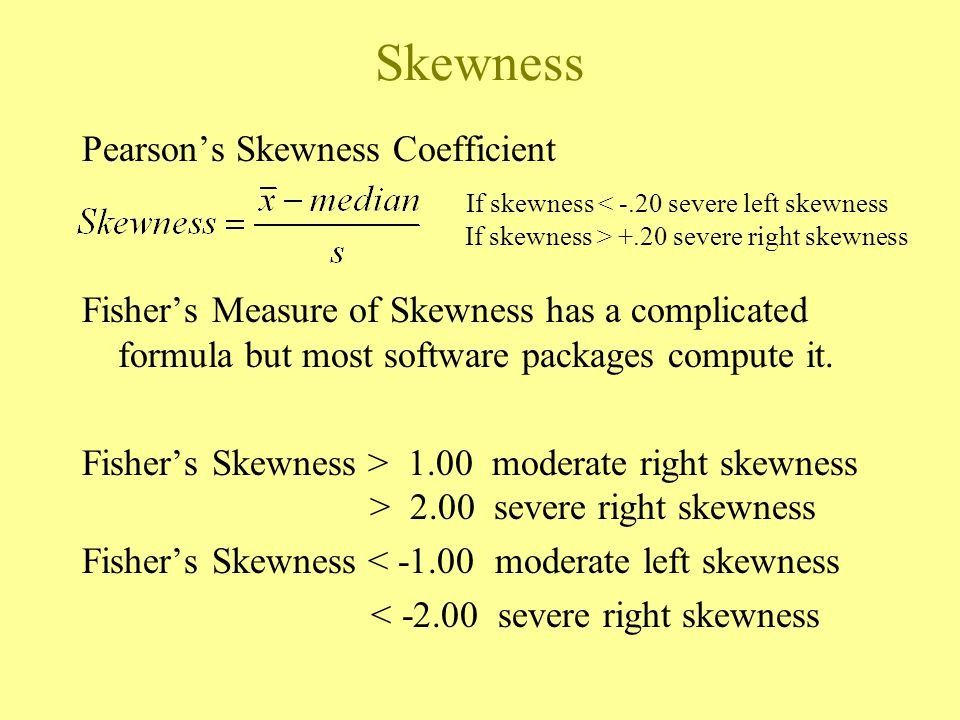Image source

Example:

Consider the following 10-number sequence that represents the scores of a competitive exam.
X = [54, 73, 59, 98, 68, 45, 88, 92, 75, 96]

By calculating the mean of X, we can get: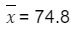Solving it with the skewness formula: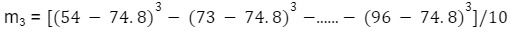The Fisher-Pearson Coefficient of Skewness is equal to 0.745631. You can see that there is a positive skew in the data.
Another way of checking is to look for the mode, median, and mean of these values.

## Kurtosis

Kurtosis is a statistical term that characterizes frequency distribution. Aside from determining if a distribution is heavy-tailed, it also provides insight into the shape of the frequency distribution.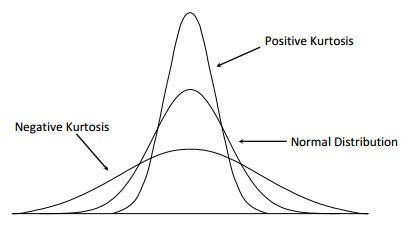Image source

Kurtosis of a normal distribution is equal to 3. When the kurtosis is less than 3, it is known as platykurtic, and when it is greater than 3, it is leptokurtic. If it is leptokurtic, it will signify that it produces outliers rather than a normal distribution.

### Calculating kurtosis

The measure of kurtosis is calculated as the fourth standardized moment of distribution. Here are the steps to follow to understand the calculation.
The Kth moment of the distribution is calculated as: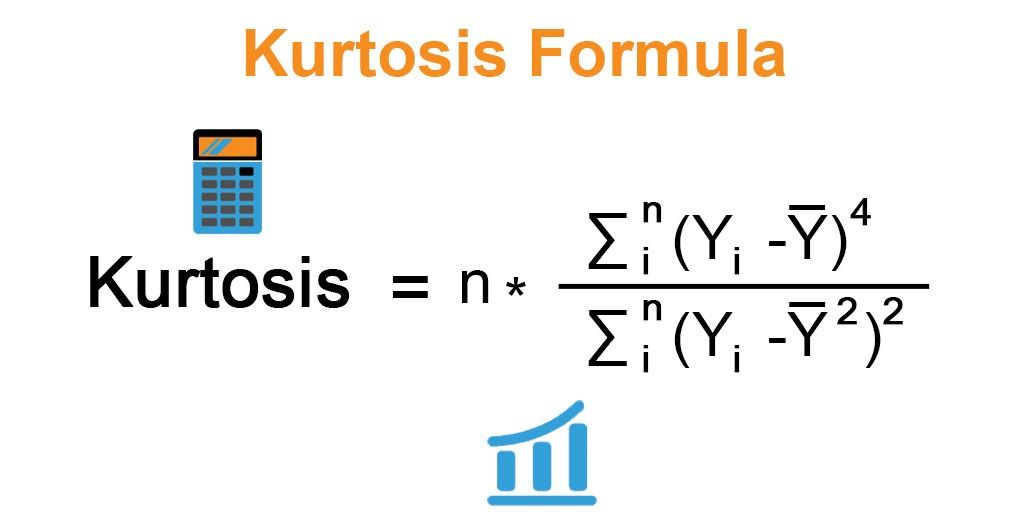Image source

As we already know, skewness is the fourth moment of a distribution. The second moment of a distribution is its variance which will help simplify the equation:Image source

Example:

We again consider a sequence of 10 numbers that represent the scores of a competitive exam. X = [54, 73, 59, 98, 68, 45, 88, 92, 75, 96]

By calculating the mean of X, we can get:You can use this value in the kurtosis formula to get the final answer.

## Calculating skewness and kurtosis in Python

Step 1: Importing the SciPy Library

SciPy Library is an open-source science library that provides in-built functions for calculating skewness and kurtosis. You can import it with the following code:

`# importing`
`SciPy`
`import SciPy`

Step 2: Creating a dataset

The next step is to create a dataset. The code below shows how.

`# creating a data set`
`dataset = [10, 25, 14, 26, 35, 45, 67, 90, 40, 50, 60, 10, 16, 18, 20]`

Step 3: Computing skewness

Use the following syntax to calculate the skewness by using the in-built skew() function.

`spicy.stats.skew(array, axis = 0, bias = True)`

where array represents the input object that contains the elements, axis signifies the axis along which we want to find the skewness value, and bias = True or False, based on the calculations that are determined upon the statistical bias.

The skewness value of the dataset will be along the axis with this return type. It will signify that the distribution will be positively skewed.

Step 4: Computing kurtosis

Calculate the kurtosis with the help of the in-built kurtosis() function using the syntax below:

`spicy.stats.kurtosis(array, axis = 0, fisher = True, bias = True)`

where the array is the input object that has the elements, and the axis represents the axis along with the kurtosis value that needs to be measured.

Fisher = True when normal is 0.0. It will be False when the normal is 3.0. Bias is True or False, based on statistical bias.

The value of kurtosis for the dataset will be the return type. It will signify that the distribution will have more values in the outputs when compared to the normal distribution.

## Measures of central tendency

The existence of random causes that influence every known variable on earth is normal. But what happens if a process comes under the influence of significant causes? This will modify the shape of the distribution and that’s when we need a measure like skewness to capture it.

The image below shows a normal distribution, which is a symmetrical graph with all measures of central tendency in the middle.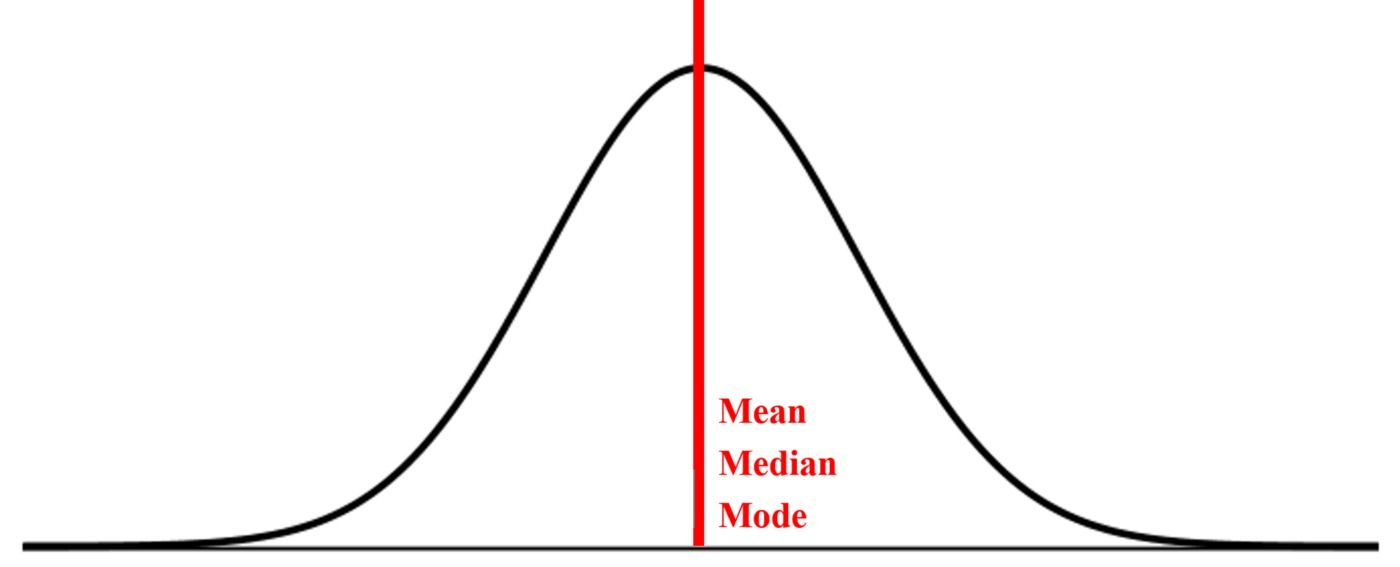Image source

However, if we find an asymmetrical distribution, we need to analyze how to detect its extent. The graph below shows the measures of central tendency.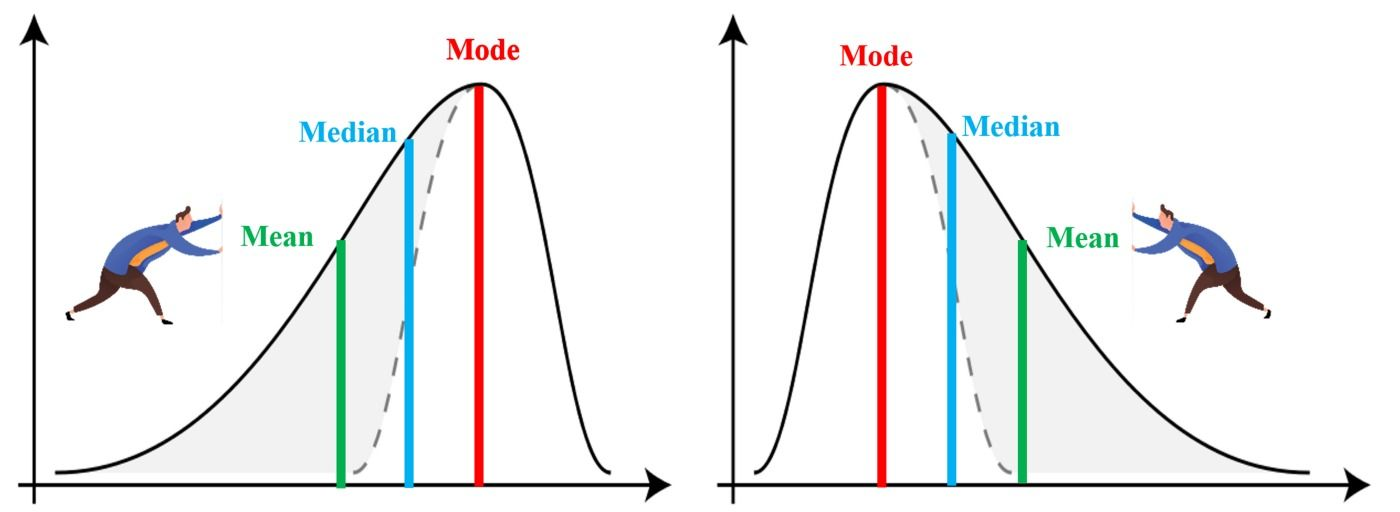Image source

Understanding how central tendency measures spread when the normal distribution is distorted is important. In the figure above, the left graph has its tail towards the left, so it is negatively skewed, while the right graph has its tail towards its right, so it is positively skewed.

We should derive a measure that will capture the horizontal distance between mode and mean. It’s important to remember that the higher the skewness, the farther apart these measures will be.

The formula for skewness is as below: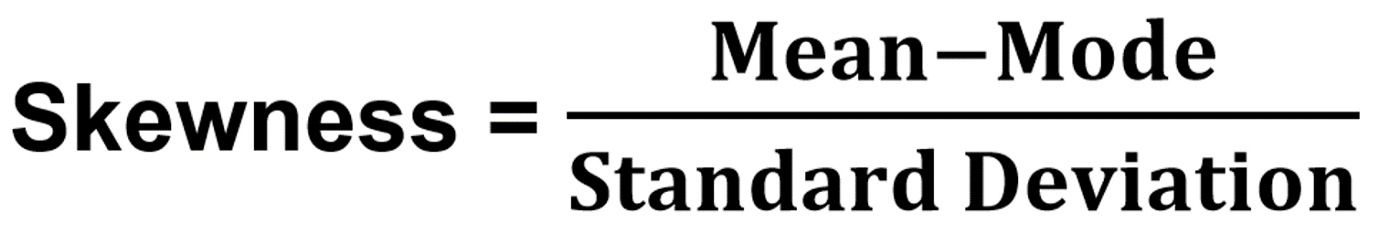With division by standard deviation, we can enable the relative comparison among distributions on the same scale. Mode calculations for small datasets are not important, so arrive at a robust formula for skewness and replace mode with the derived calculation from the mean and median.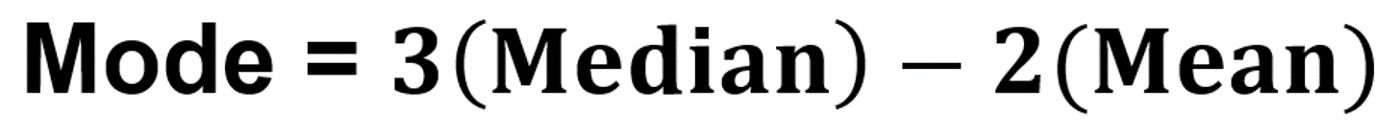Replacing the mode value in the formula, we get: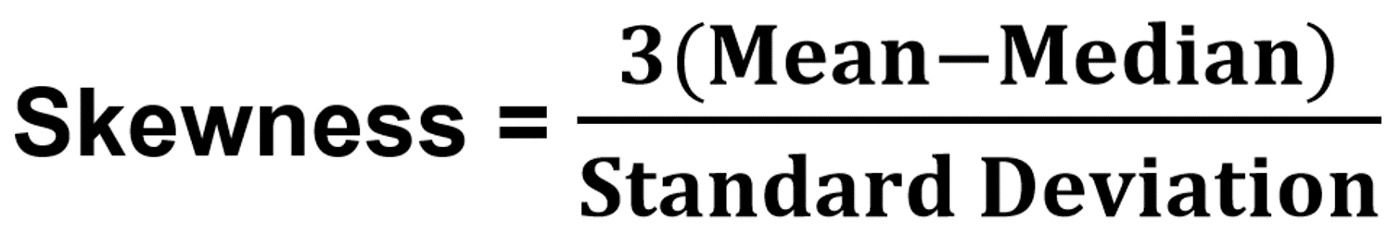You should consider pulling the normal distribution curve from the top and understand the shape of the impact. There are two things to notice: the peak of the curve and the tails. The kurtosis measure will be responsible for capturing this.

The kurtosis calculation is complex so it’s important to stick to the concept for visual clarity.

To reiterate, a normal distribution has a kurtosis 3 (known as mesokurtic). The distributions that are greater than 3 are leptokurtic, and those lower than 3 are platykurtic. The higher the values, the higher the peak, and kurtosis will range from 1 to infinity.

We can calculate excess kurtosis by keeping zero as a reference for normal distribution with the formula below: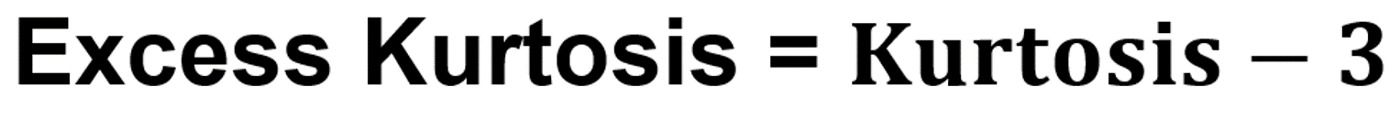The horizontal pull distortion of a normal distribution curve will be captured by the skewness measure. Meanwhile, the vertical distortion will be captured by the kurtosis measure. The impact of outliers that dominates the kurtosis effect has its roots of proof sitting in the fourth-order moment formula.### Press

What's up with Turing? Get the latest news about us here.### Blog